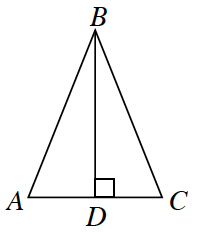Home > CCG > Chapter 9 > Lesson 9.1.1 > Problem9-13

9-13.

Multiple Choice: What information would you need to know about the diagram below in order to prove that $ΔABD ≅ ΔCBD$ by $SAS ≅$? Homework Help ✎1. $AD ≅ CD$

1. $AB ≅ CB$

1. $∠A ≅ ∠C$

1. $∠ABC ≅ ∠CBD$

1. None of these

(a)